# NCERT Solutions Class 10 Science Chapter 13 Magnetic Effect Of Current

Ncert Solutions for class 10 subject Science Chapter 13 Magnetic Effect Of Currentin pdf Best Free NCERT Solutions for class 1 to 12 in pdf NCERT Solutions, cbse board, Science, ncert Solutions for Class 10 Science, class 10 Science ncert solutions, Magnetic Effect Of Current, Class 10, ncert solutions chapter 13 Magnetic Effect Of Current, class 10 Science, class 10 Science ncert solutions, Science ncert solutions class 10, Ncert Solutions Class 10 Science Chapter 13 Magnetic Effect Of Current

## In text Question Chapter 13 Class 10 Science Magnetic Effect Of Current Ncert Solutions

In text Questions of Page No. 224

Q. No 1: Why does a compass needle get deflected when brought near a bar magnet?
Ans:
The needle of a compass is a small magnet. That’s why when a compass needle is brought near a bar magnet, its magnetic field lines interact with that of the bar magnet. Hence, a compass needle gets deflected.

In text Questions of Page No. 228

Q. No 1: Draw magnetic field lines around a bar magnet.
Ans:
Following diagram shows the magnetic field lines around a bar magnet.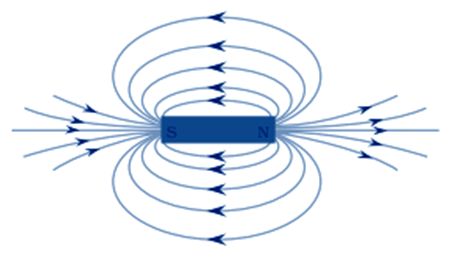Q. No 2: List the properties of magnetic lines of force.
Ans:
The properties of magnetic lines of forces are:
1) The direction of magnetic field lines outside the magnet sis from North Pole to South Pole.
2) The direction of magnetic field lines inside the magnet is from south pole to the north pole.
3) No two magnetic field lines intersect each other.

Q. No 3: Why don’t two magnetic lines of force intersect each other?
Ans:
The two magnetic field lines do not intersect each other because if they do it means at the point of intersect the compass needle is showing two different directions which is not possible.

In text Questions of Page No. 229

Q. No 1: Consider a circular loop of wire lying in the plane of the table. Let the current pass through the loop clockwise. Apply the right-hand rule to find out the direction of the magnetic field inside and outside the loop.
Ans:
In the following diagram the current is flowing clockwise. If we are applying right hand thumb rule to the left side of the loop then the direction of magnetic field lines inside the loop are going into the table while outside the loop they are coming out of the table. If we are applying right hand thumb rule to the right side of the loop then the direction of magnetic field lines inside the loop are again going into the table while outside the loop they are coming out of the table.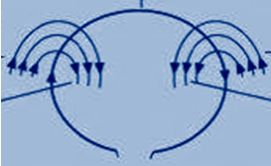Q. No 2: The magnetic field in a given region is uniform. Draw a diagram to represent it.
Ans:
A uniform magnetic field is represented by parallel lines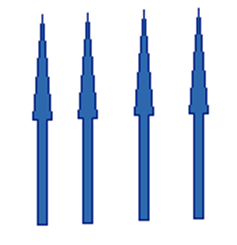Q. No 3: Choose the correct option.
The magnetic field inside a long straight solenoid-carrying current
(a) is zero
(b) decreases as we move towards its end
(c) increases as we move towards its end
(d) is the same at all points
Ans:
(d) The magnetic field inside a long straight current-carrying solenoid is uniform which is represented by parallel lines. Hence it is same at all points.

In text Questions of Page No. 231

Q. No 1: Which of the following property of a proton can change while it moves freely in a magnetic field?
(a) mass , (b)speed ,(c) velocity, (d) momentum
Ans:
velocity and momentum

Q. No 2: In Activity 13.7 (page: 230), how do we think the displacement of rod AB will be affected if (i) current in rod AB is increased: (ii) a stronger horse-shoe magnet is used: and (iii) length of the rod AB is increased?
Ans:
(i) If the current in the rod is increased then rod will be deflected with greater force.
(ii) If a stronger horse-shoe magnet is used then also rod will be deflected with greater force due to the increase in magnetic field.
(iii) If the length of the rod AB is increased.

Q. No 3: A positively-charged particle (alpha-particle) projected towards west is deflected towards north by a magnetic field. The direction of magnetic field is
(a) towards south (b) towards east
(c) downward (d) upward
Ans: (d)
The direction of the magnetic field can be determined by the Fleming’s left hand rule. According to Fleming’s left hand rule if we stretch the thumb, the centre finger and the middle finger of our left hand such that they are mutually perpendicular to each other. If the centre finger gives the direction of current and middle finger points in the direction of magnetic field then the thumb points towards the direction of the force or motion of the conductor.
Here the direction of positively charged alpha particle is towards west this implies the direction of current is also towards west and the direction of magnetic force is towards north. Hence, according to Fleming’s left hand rule, the direction of magnetic field will be upwards.

In text Questions of Page No. 233

Q. No 1: State Fleming’s left-hand rule.
Ans:
According to Fleming’s left hand rule if we stretch the thumb, the centre finger and the middle finger of our left hand such that they are mutually perpendicular to each other. If the centre finger gives the direction of current and middle finger points in the direction of magnetic field then the thumb points towards the direction of the force or motion of the conductor.

Q. No 2: What is the principle of an electric motor?
Ans:
The principle of an electric motor is based on the magnetic effect of electric current. A current-carrying loop experiences a force and rotates when placed in a magnetic field. The direction of rotation of the loop is according to the Fleming’s left-hand rule.

Q. No 3: What is the role of the split ring in an electric motor?
Ans:
The split ring in the electric motor also known as a commutator reverses the direction of current flowing through the coil after every half rotation of the coil. Due to this the coil continues to rotate in the same direction.

In text Questions of Page No. 236

Q. No 1: Explain different ways to induce current in a coil.
Ans:
The different ways to induce current in a coil are as follows:
(i) Moving a magnet towards a coil sets up a current in the coil circuit,
(ii) If a coil is moved rapidly between the two poles of a horse-shoe magnet, then an electric current is induced in the coil.

In text Questions of Page No. 237

Q. No 1: State the principle of an electric generator.
Ans:
Electric generator works on the principle of electromagnetic induction. Electricity is generated by rotating a coil inside magnetic field.

Q. No 2: Name some sources of direct current.
Ans:
Some sources of direct current are cell, battery and DC generator, etc.

Q. No 3: Which sources produce alternating current?
Ans:
hydroelectric power plants, AC generators produce alternating current.

Q. No 4: Choose the correct option.
A rectangular coil of copper wires is rotated in a magnetic field. The direction of the induced current changes once in each
(a) two revolutions (b) one revolution
(c) half revolution (d) one-fourth revolution
Ans:

(c) When a rectangular coil of copper is rotated in a magnetic field, the direction of the induced current in the coil changes in every half rotation.

In text Questions of Page No. 238

Q. No 1: Name two safety measures commonly used in electric circuits and appliances.
Ans:
Two safety measures commonly used in electric circuits and appliances are
(i) Electric Fuse: An electric fuse is connected in series it protects the circuit from overloading and prevents it from short circuiting.
(ii) Proper earthing of all electric circuit in which any leakage of current in an electric appliance is transferred to the ground and people using the appliance do not get the shock.

Q. No 2: An electric oven of 2 kW is operated in a domestic electric circuit (220 V) that has a current rating of 5 A. What result do you expect? Explain.
Ans:
In this problem we have
Power of the oven (P) = 2 kW = 2000 W
Potential Difference (V) = 220 V
We have P = VI
⇒I=P/V
= 2000/220
= 9.09A
Here the current drawn by the electric oven is 9.09 A, which exceeds the safe limit of the circuit that is 5A. Therefore electric fuse will melt and break the circuit.

Q. No 3: What precaution should be taken to avoid the overloading of domestic electric circuits?
Ans:
Following precautions should be taken to avoid the overloading of domestic circuits:
(a) Do not use too many appliances at the same time.
(b) Use the appliances within the safe limit of electric circuit.
(c) Do not connect too many appliances in a single socket.
(c) Fuse should be connected in series in the circuit to protect overloading and short circuiting.

## Exercise Chapter 13 Class 10 Science Magnetic Effect Of Current Ncert Solutions

Q. No 1: Which of the following correctly describes the magnetic field near a long straight wire?
(a) The field consists of straight lines perpendicular to the wire
(b) The field consists of straight lines parallel to the wire
(c) The field consists of radial lines originating from the wire
(d) The field consists of concentric circles centred on the wire
Ans:
According to right hand thumb rule the magnetic field lines, produced around a straight current-carrying conductor, are concentric circles.

Q. No 2: The phenomenon of electromagnetic induction is
(a) the process of charging a body
(b) the process of generating magnetic field due to a current passing through a coil
(c) producing induced current in a coil due to relative motion between a magnet and the coil
(d) the process of rotating a coil of an electric motor
Ans:
The option (c) is correct that is when a straight coil and a magnet are moved relative to each other then a current is induced in the coil.

Q. No 3: The device used for producing electric current is called a
(a) Generator, (b) galvanometer, (c) ammeter and (d) motor
Ans: (a)
Electric current is produced by electric generator which converts mechanical energy into electricity.

Q. No 4: The essential difference between an AC generator and a DC generator is that
(a) AC generator has an electromagnet while a DC generator has permanent magnet.
(b) DC generator will generate a higher voltage.
(c) AC generator will generate a higher voltage.
(d) AC generator has slip rings while the DC generator has a commutator.
Ans:
The option (d) is correct that an AC generator has slip rings while a DC generator has commutator.

Q. No 5: At the time of short circuit, the current in the circuit
(a) reduces substantially
(b) does not change
(c) increases heavily
(d) vary continuously.
Ans:
The option (c) is correct. At the time of short circuiting then the amount of current that is flowing in the circuit increases.

Q. No 6: State whether the following statements are true or false.
(a) An electric motor converts mechanical energy into electrical energy.
(b) An electric generator works on the principle of electromagnetic induction.
(c) The field at the centre of a long circular coil carrying current will be parallel straight lines.
(d) A wire with a green insulation is usually the live wire of an electric supply.
Ans:

(a) False: An electric motor converts electrical energy into mechanical energy.
(b) True: A generator is an electric device that generates electricity by rotating a coil in a magnetic field. It works on the principle of electromagnetic induction.
(c) True: A long circular coil is a long solenoid. The magnetic field lines inside the solenoid are parallel lines.
(d) False:Live wire has red insulation cover, whereas earth wire has green insulation colour in the domestic circuits.

Q. No 7: List three sources of magnetic fields.
Ans:
Three sources of magnetic fields are:
(a) Permanent magnet
(b) Electromagnet
(c) Current-carrying conductor

Q. No 8: How does a solenoid behave like a magnet? Can you determine the north and south poles of a current-carrying solenoid with the help of a bar magnet? Explain.
Ans:
Solenoid is coil having n number of turns of insulated copper wire. Magnetic field lines are produced around the solenoid when a current is passed through it. The magnetic field produced by it is similar to the magnetic field of a bar magnet. The field lines produced in a current-carrying solenoid is shown in the following figure.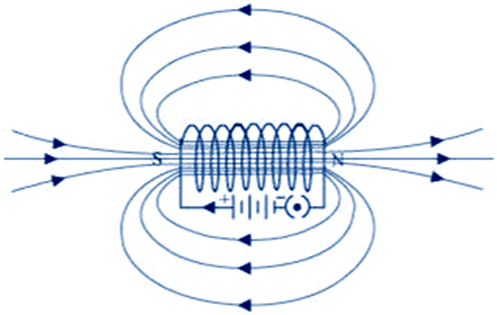When the north pole of a bar magnet is brought near to the end connected to the negative terminal of the battery, then the solenoid repels the bar magnet. It means the end of solenoid which is connected to the negative terminal of the battery behaves as north pole as like poles repel each other similarly the other and behaves as a south pole.

Q. No 9: When is the force experienced by a current-carrying conductor placed in a magnetic field largest?
Ans:
The force experienced by a current-carrying conductor is largest when the directions of magnetic field and electric current are perpendicular to each other.

Q. No 10: Imagine that you are sitting in a chamber with your back to one wall. An electron beam, moving horizontally from back wall towards the front wall, is deflected by a strong magnetic field to your right side. What is the direction of magnetic field?
Ans:
The direction of magnetic field is given by Fleming’s left hand rule. The direction of electric current will be from front wall to back wall as it is opposite to the direction of electron. Now since the direction of force is towards the right side. Hence by applying Fleming’s left hand rule, we can conclude that the magnetic field inside the chamber is in downward direction.

Q. No 11: Draw a labelled diagram of an electric motor. Explain its principle and working. What is the function of a split ring in an electric motor?
Ans:
An electric motor is a device which converts electrical energy into mechanical energy. The principle behind the electric motor is based on Fleming’s left hand rule.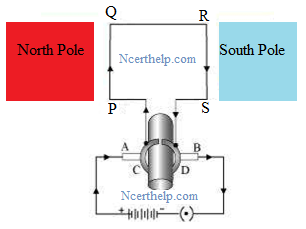Working: When a current is allowed to flow through the coil PQRS the coil starts rotating anti-clockwise. This happens because a downward force acts on length PQ and at the same time, an upward force acts on length RS. As a result, the coil rotates anti-clockwise.
Current in the length PQ flows from P to Q and the magnetic field acts from left to right, normal to length PQ. Therefore, according to Fleming’s left hand rule, a downward force acts on the length PQ. Similarly at an upward force acts on the length RS. These two forces cause the coil to rotate anti-clockwise.
When coil complete half rotation, the position of PQ and RS interchange. The half-ring D comes in contact with brush A and half-ring C comes in contact with brush B. therefore the direction of current in the coil PQRS gets reversed. Now the current flows through the coil in the direction SRQP.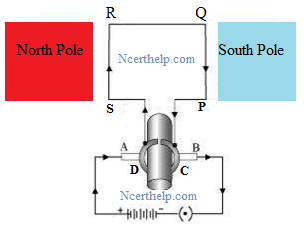The direction of current through the coil PQRS reverse after every half rotation. As a result, the coil rotates in same direction. The split rings help to reverse the direction of current in the circuit. These are called the commutator.

Q. No 12: Name some devices in which electric motors are used?
Ans: Electric motors are used in:
Water pumps
Electric fans
Electric mixers
Washing machines e.t.c

Q. No 13: A coil of insulated copper wire is connected to a galvanometer. What will happen if a bar magnet is (i) pushed into the coil, (ii) withdrawn from inside the coil, (iii) held stationary inside the coil?
Ans: (i) when magnet is pushed near a bar magnet then it induces current due to electromagnetic induction.
(ii) When the bar magnet is withdrawn from inside the coil of the insulated copper wire again the current is induced in the coil but this time it is in reverse direction.
(iii) When a bar magnet is held stationary inside the coil then no current is induced that’s why galvanometer will show no deflection.

Q. No 14: Two circular coils A and B are placed closed to each other. If the current in the coil A is changed, will some current be induced in the coil B? Give reason.
Ans: If the current in the coil A is changed then some current will definitely be induced in the coil B. When the current in coil A is changed, the magnetic field associated with it also changes. As a result, the magnetic field around coil B also changes. This change in magnetic field lines around coil B induces an electric current in it. This process is known electromagnetic induction.

Q. No 15: State the rule to determine the direction of a (i) magnetic field produced around a straight conductor-carrying current, (ii) force experienced by a current-carrying straight conductor placed in a magnetic field which is perpendicular to it, and (iii) current induced in a coil due to its rotation in a magnetic field.
Ans: (i) Right hand thumb rule
(ii) Fleming’s left hand rule
(iii) Fleming’s right hand rule

Q. No 16: Explain the underlying principle and working of an electric generator by drawing a labelled diagram. What is the function of brushes?
Ans: An electric generator converts mechanical energy into electrical energy. The principle behind the electric motor is based on Fleming’s right hand rule.
when a coil of insulated copper wire is forced to rotate inside a magnetic field then electric current is induced. The following figure shows circuit diagram of a simple AC generator. If axle X is rotated clockwise, then the length PQ moves upwards and the length RS moves downwards.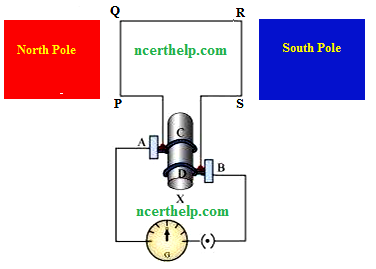Since the lengths PQ and RS are moving in a magnetic field, a current will be induced due to electromagnetic induction. Length PQ is moving upwards and the magnetic field acts from left to right. Hence, according to Fleming’s right hand rule, the direction of induced current will be from P to Q. Similarly, the direction of induced current in the length RS will be from R to S. The direction of current in the coil is PQRS. Hence, the galvanometer shows a deflection in a particular direction. After half a rotation, length PQ starts moving down whereas length RS starts moving upward. The direction of the induced current in the coil gets reversed as SRQP. As the direction of current gets reversed after each half rotation, the produced current is called an alternating current (AC). To get a unidirectional current, instead of two slip rings, two split rings are used, as shown in the following figure. In this arrangement, brush A always remains in contact with the length of the coil that is moving up whereas brush B always remains in contact with the length that is moving down. The split rings C and D act as a commutator.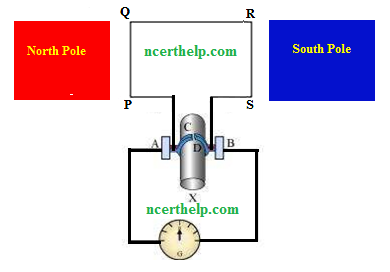The direction of current induced in the coil will be PQRS for the first half and SRQP in the second half of the rotation. Therefore a unidirectional current is produced from the generator called DC generator

Q. No 17: When does an electric short circuit occur?
Ans: If the insulation of the wires used in the circuit is damaged or the appliance used is faulty due to which the live wire and the neutral wire comes in direct contact as a result current in the circuit rises and the short circuit occurs.

Q. No 18: What is the function of an earth wire? Why is it necessary to earth metallic appliances?
Ans: The metallic body of electric appliances is connected to the earth wire so that any leakage of electric current is transferred to the ground. This prevents severe electric shock to the user. That is why earthing of the electrical appliances is necessary.

### NCERT Books Free Pdf Download for Class 5, 6, 7, 8, 9, 10 , 11, 12 Hindi and English Medium

 Mathematics Biology Psychology Chemistry English Economics Sociology Hindi Business Studies Geography Science Political Science Statistics Physics Accountancy